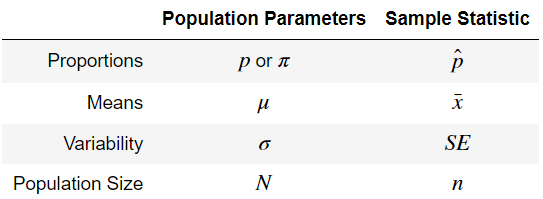# Samples, Populations, and their Symbols

### Terminology

Samples come from populations, and represent a smaller subset of all possible values.

• e.g. If you email 100 clients at random from a list of 10,000 clients.

Statistics describe samples whereas parameters describe populations (alliteration, FTW)

• e.g. The “average age of all clients” vs “average age of the 100 clients we selected”

### Symbols

Generaly, Greek tends to mean population, whereas things with hats tend to mean sample.

# cheating because rendering table w/ latex
# in jupyter and hugo is a headache
from IPython.display import Image
Image(filename='../images/symbol_table.png')## Calculating Sample Statistics

### Proportions

Sample Proportion

$$\hat{p} = \frac{\text{Number of successes}}{\text{sample size}}= \frac{X}{n}$$

Standard Error

$$SE_\hat{p} = \sqrt{\frac{\% successes \times \% failures}{\text{sample size}}} = \sqrt{\frac{\hat{p}(1 - \hat{p})}{n}}$$

### Means

Sample Mean

$$\bar{X} = \frac{\text{sum of all observations}}{\text{sample size}} = \frac{x_1 + x_2 + \dots + x_n}{n}$$

Standard Error

$$SE_\bar{X} = \frac{\text{sample std dev}}{\text{factor of sample size}} = \frac{s_x}{\sqrt{n}}$$

### A Note on the sqrt(n)’s

Both Standard Errors listed above are measures of variation on the center statistic of the distribution

Let’s do a quick derivation on why this works.

If x1, x2, … , xn are independent from a population w/ mean and stdev $\mu, \sigma$ then the variance of their total is

$$n\sigma^{2}$$

And because the sample mean is expressed as

$$\bar{X} = \frac{x_1, x_2, \dots, x_n}{n}$$

We can substitute that into the variance calculation

$$Var(\bar{X}) = Var(\frac{1}{n}\sum\limits_{i=1}^{n}X_i)$$

$$= \frac{1}{n^2}\sum\limits_{i=1}^{n}Var(X_i)$$

$$= \frac{1}{n^2}n^2\sigma^2\frac{1}{n}$$

$$= \frac{\sigma^2}{n}$$

Thus, the standard deviation of this becomes $\frac{\sigma}{\sqrt{n}}$